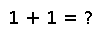# enumerate

enumerate(iter)

An iterator that yields `(i, x)` where `i` is an index starting at 1, and `x` is the `i`th value from the given iterator. It's useful when you need not only the values `x` over which you are iterating, but also the index `i` of the iterations.

``````julia> a = ["a", "b", "c"];

julia> for (index, value) in enumerate(a)
println("\$index \$value")
end
1 a
2 b
3 c``````

## Examples

``````julia> a = ["a", "b", "c"];
for (index, value) in enumerate(a)
println("\$index \$value")
end
1 a
2 b
3 c``````
``````julia> foo = [-1, -2, -3];
julia> for (index, value) in enumerate(foo)
println("Element at index \$index : \$value")
end
Element at index 1 : -1
Element at index 2 : -2
Element at index 3 : -3``````

Checking you are not a robot: